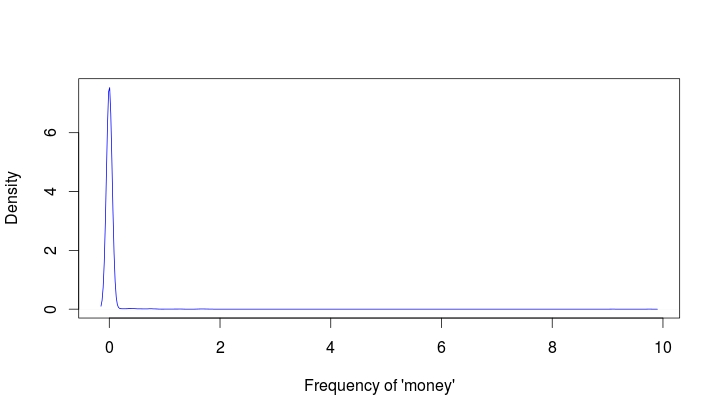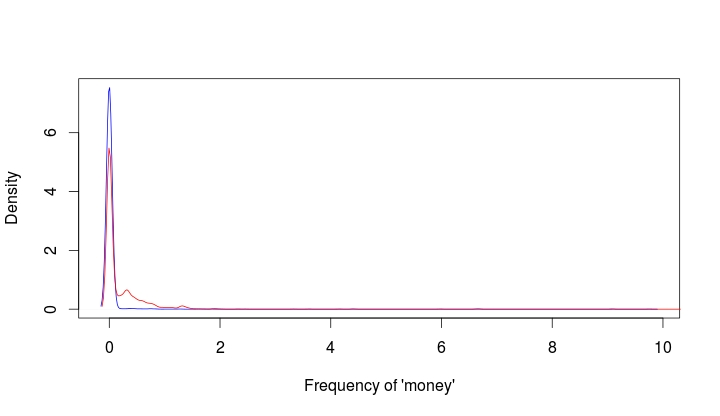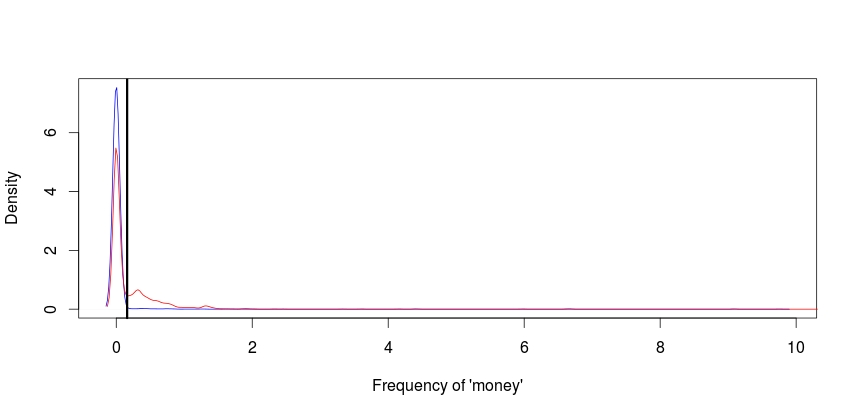# Simple Prediction

Prediction is one of the key concepts of machine learning. Machine learning is a field of study that is focused on the development of algorithms that can be used to make predictions.Anyone who has shopped online at has experienced machine learning. When you make a purchase at an online store, the website will recommend additional purchases for you to make. Often these recommendations are based on whatever you have purchased or whatever you click on while at the site.

There are two common forms of machine learning, unsupervised and supervised learning. Unsupervised learning involves using data that is not cleaned and labeled and attempts are made to find patterns within the data. Since the data is not labeled, there is no indication of what is right or wrong

Supervised machine learning is using cleaned and properly labeled data. Since the data is labeled there is some form of indication whether the model that is developed is accurate or not. If the is incorrect then you need to make adjustments to it. In other words, the model learns based on its ability to accurately predict results. However, it is up to the human to make adjustments to the model in order to improve the accuracy of it.

In this post, we will look at using R for supervised machine learning. The definition presented so far will make more sense with an example.

The Example

We are going to make a simple prediction about whether emails are spam or not using data from kern lab.

The first thing that you need to do is to install and load the “kernlab” package using the following code

```install.packages("kernlab")
library(kernlab)```

If you use the “View” function to examine the data you will see that there are several columns. Each column tells you the frequency of a word that kernlab found in a collection of emails. We are going to use the word/variable “money” to predict whether an email is spam or not. First, we need to plot the density of the use of the word “money” when the email was not coded as spam. Below is the code for this.

```plot(density(spam\$money[spam\$type=="nonspam"]),
col='blue',main="", xlab="Frequency of 'money'")

```

This is an advance R post so I am assuming you can read the code. The plot should look like the following.As you can see, money is not used to frequently in emails that are not spam in this dataset. However, you really cannot say this unless you compare the times ‘money’ is labeled nonspam to the times that it is labeled spam. To learn this we need to add a second line that explains to us when the word ‘money’ is used and classified as spam. The code for this is below with the prior code included.

```plot(density(spam\$money[spam\$type=="nonspam"]),
col='blue',main="", xlab="Frequency of 'money'")
lines(density(spam\$money[spam\$type=="spam"]), col="red")```

Your new plot should look like the followingIf you look closely at the plot doing a visual inspection, where there is a separation between the blue line for nonspam and the red line for spam is the cutoff point for whether an email is spam or not. In other words, everything inside the arc is labeled correctly while the information outside the arc is not.

The next code and graph show that this cutoff point is around 0.1. This means that any email that has on average more than 0.1 frequency of the word ‘money’ is spam. Below is the code and the graph with the cutoff point indicated by a black line.

```plot(density(spam\$money[spam\$type=="nonspam"]),
col='blue',main="", xlab="Frequency of 'money'")
lines(density(spam\$money[spam\$type=="spam"]), col="red")
abline(v=0.1, col="black", lw= 3)```Now we need to calculate the accuracy of the use of the word ‘money’ to predict spam. For our current example, we will simply use in “ifelse” function. If the frequency is greater than 0.1.

We then need to make a table to see the results. The code for the “ifelse” function and the table are below followed by the table.

```predict<-ifelse(spam\$money > 0.1, "spam","nonspam")
table(predict, spam\$type)/length(spam\$type)```
```predict       nonspam        spam
nonspam 0.596392089 0.266898500
spam    0.009563138 0.127146273```

Based on the table that I am assuming you can read, our model accurately calculates that an email is spam about 71% (0.59 + 0.12) of the time based on the frequency of the word ‘money’ being greater than 0.1.

Of course, for this to be true machine learning we would repeat this process by trying to improve the accuracy of the prediction. However, this is an adequate introduction to this topic.